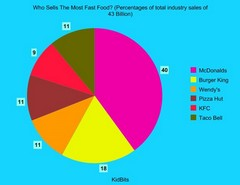# SAT Mathematics Level 2: Help and Review Final Exam

Free Practice Test Instructions:

Choose your answer to the question and click 'Continue' to see how you did. Then click 'Next Question' to answer the next question. When you have completed the free practice test, click 'View Results' to see your results. Good luck!

#### Question 2 2. Let the set A = {2, 4, 6, 8} Let set B = {1, 3, 5, 7} Find.#### Question 6 6. This graph corresponds to which inequality?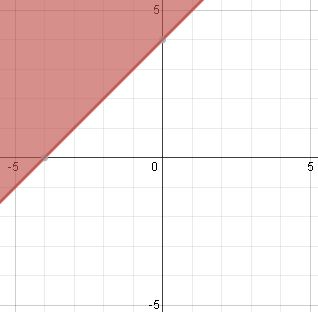#### Question 7 7. In the pictured triangle, side a is 17 and side c is 20. If ∠B is 19 degrees, then approximately how long is side b? Round the value of the cosine to the nearest whole number.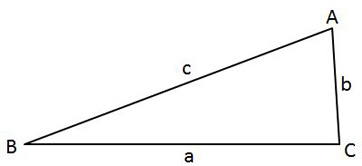#### Question 8 8. During the first four Summer Olympic games attended by the United States, these medal counts were awarded. Calculate the mean number of medals won during these Olympic Games. (Round it to the nearest whole number.)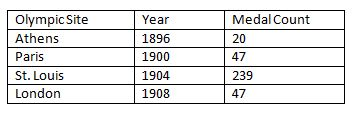#### Question 9 9. What is the complex conjugate of the expression below?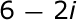#### Question 15 15. Looking at the pie chart 'Who Sells The Most Fast Food?', how many sectors can be determined?